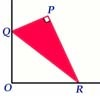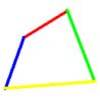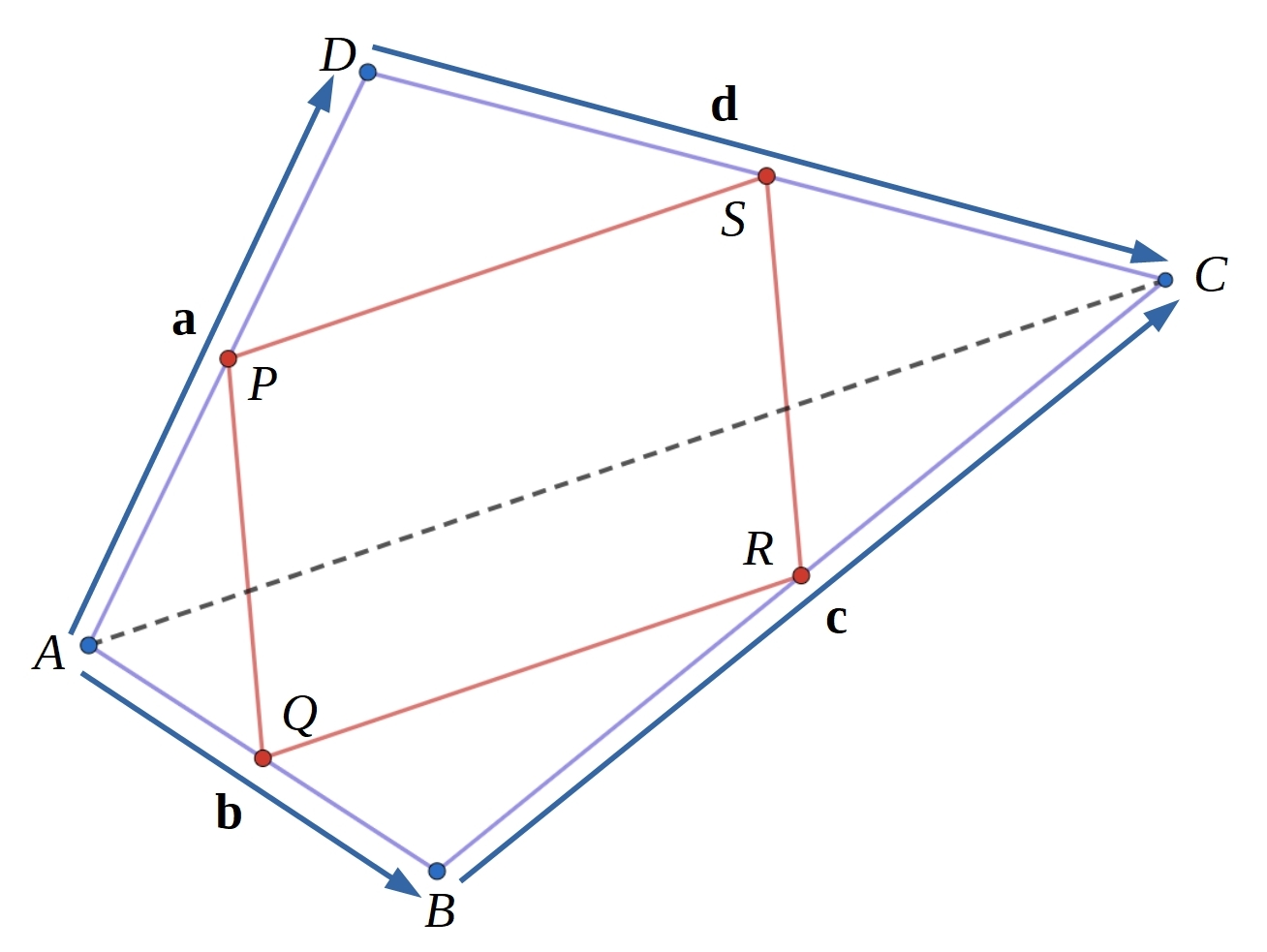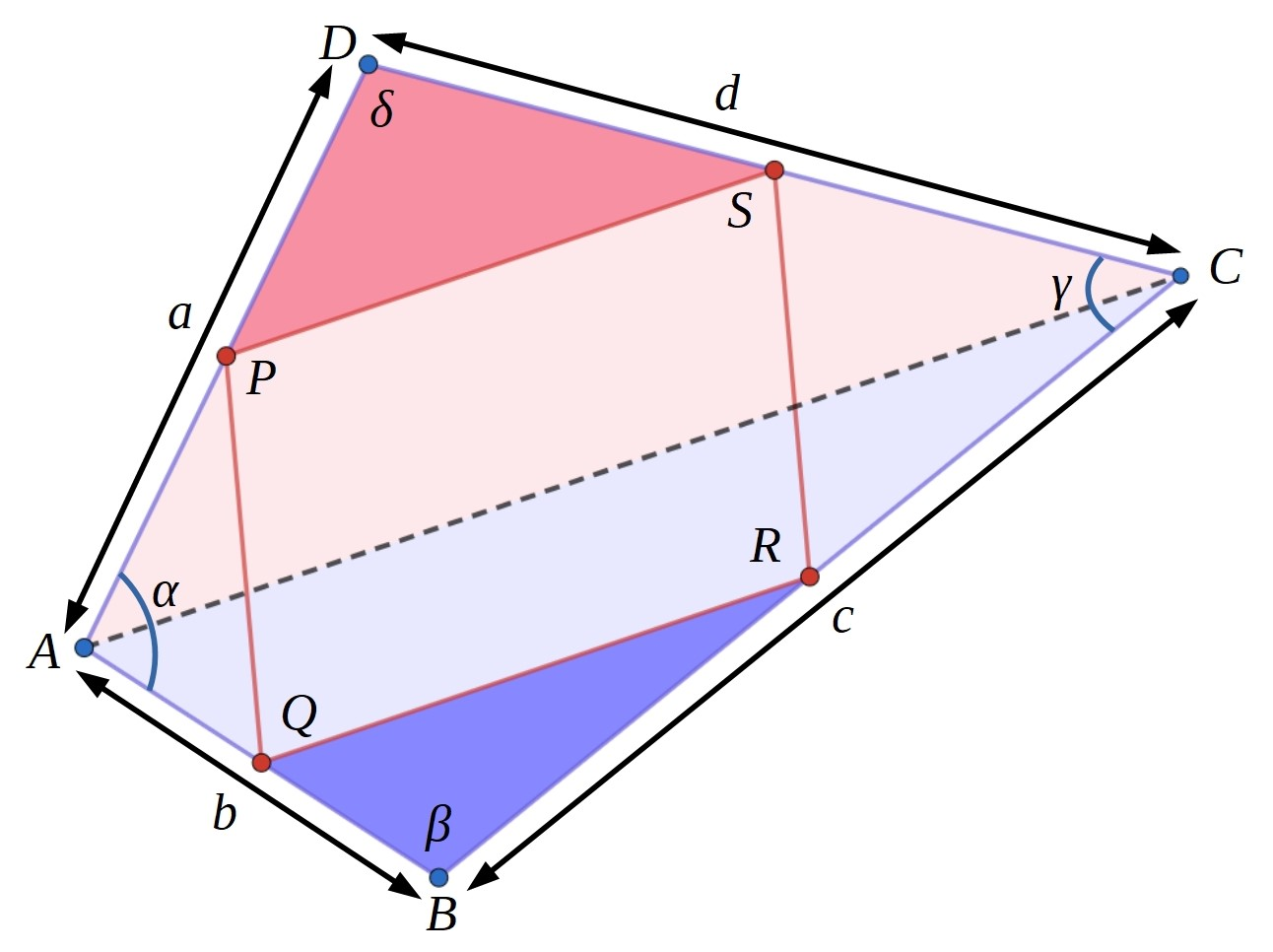#### You may also like### Set Square

A triangle PQR, right angled at P, slides on a horizontal floor with Q and R in contact with perpendicular walls. What is the locus of P?Four rods are hinged at their ends to form a convex quadrilateral. Investigate the different shapes that the quadrilateral can take. Be patient this problem may be slow to load.### Biggest Bendy

Four rods are hinged at their ends to form a quadrilateral. How can you maximise its area?

##### Age 14 to 18Challenge Level

Printable dotty paper

Draw any convex quadrilateral and find the midpoints of the four edges.
Join the midpoints to form a new quadrilateral.

A convex quadrilateral is one where all of the angles are less than $180^{\circ}$.  Alternatively you can use the definition that both diagonals lie inside the shape.

Try it a few times starting with different convex quadrilaterals.

What do you notice about the new quadrilaterals formed by joining the midpoints?
Does this always happen?

Can you find a counter-example?
Can you make a conjecture?
You may wish to use the GeoGebra interactivity to explore what happens as you move points A, B, C and D.

Is the area of PQRS always the same fraction of the area of ABCD?
Can you explain why?

Once you have had a think about this, you might like to take a look at these two different proofs which have been scrambled up. Can you rearrange them back into their original order?Shape of PQRS proofArea of PQRS proof

Do these results still hold if ABCD is a concave quadrilateral?
A concave quadrilateral is one where one angle is greater than $180^{\circ}$, for example you could draw an "arrowhead" shape.

If you can find a proof which is different to the ones in our proof sorters, then please do let us know by submitting it as a solution.

We are very grateful to the Heilbronn Institute for Mathematical Research for their generous support for the development of this resource.